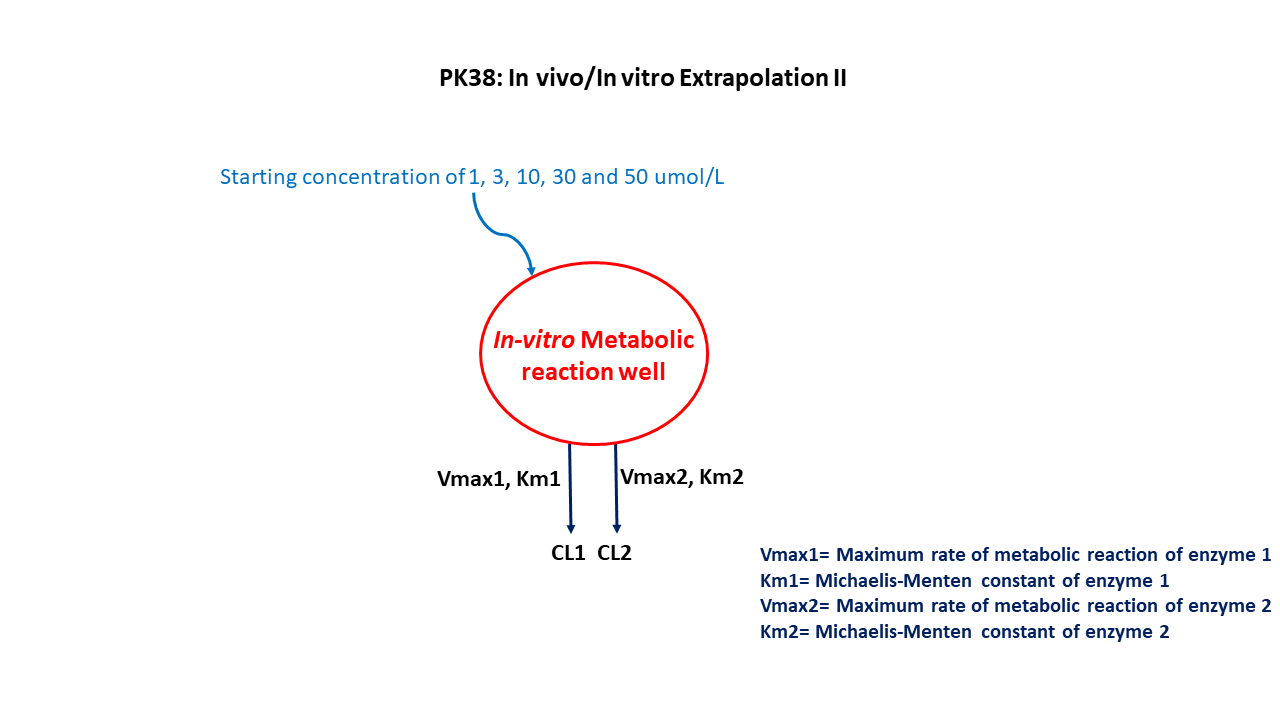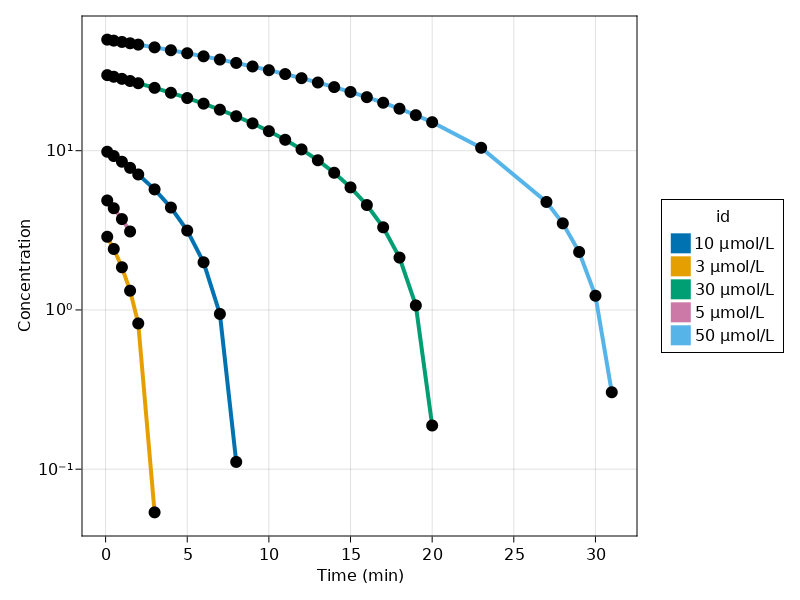# Exercise PK38 - Invitro/invivo extrapolation II

### Background

• Structural Model - Two Enzyme Model

• Route of adminstration - in vitro experiment

• Dosage regimen - 50,30,10,3,1 μmol### Learning Outcome

To analyze data based on the metabolic rate of compound using differential equations instead transforming data into rate versus concentration plot.

### Libraries

Call the "necessary" libraries to get started.

using Random
using Pumas
using PumasUtilities
using CairoMakie
using AlgebraOfGraphics
using DataFramesMeta


### Model

pk_38        = @model begin
desc = "Invitro/Invivo Extrapolation Model"
timeu = u"minute"
end

@param begin
"Maximum Metabolic Capacity of System 1 (nmol/min)"
tvvmax1  ∈ RealDomain(lower=0)
"Michelis Menten Constant of System 1 (μmol/l)"
tvkm1    ∈ RealDomain(lower=0)
"Maximum Metabolic Capacity of System 2 (nmol/min)"
tvvmax2  ∈ RealDomain(lower=0)
"Michelis Menten Constant of System 2 (μmol/l)"
tvkm2    ∈ RealDomain(lower=0)
Ω        ∈ PDiagDomain(4)
end

@random begin
η        ~ MvNormal(Ω)
end

@pre begin
Vmax1    = tvvmax1 * exp(η)
Km1      = tvkm1 * exp(η)
Vmax2    = tvvmax2 * exp(η)
Km2      = tvkm2 * exp(η)
Vmedium  = 1
end

@vars begin
VMKM    := ((Vmax1*Central/(Km1+Central))+(Vmax2*Central/(Km2+Central)))
end

@dynamics begin
Central' =  -VMKM/Vmedium
end

@derived begin
cp       = @. Central/Vmedium
"""
Observed Concentration (μmol/L)
"""
end
end

PumasModel
Parameters: tvvmax1, tvkm1, tvvmax2, tvkm2, Ω, σ_add
Random effects: η
Covariates:
Dynamical variables: Central
Derived: cp, dv
Observed: cp, dv


### Parameters

Parameters provided for simulation are as below. tv represents the typical value for parameters.

• $Vmax1$ - Maximum Metabolic Capacity of System 1 (nmol/min)

• $Km1$ - Michelis Menten Constant of System 1 (μmol/l)

• $Vmax2$ - Maximum Metabolic Capacity of System 2 (nmol/min)

• $Km2$ - Michelis Menten Constant of System 2 (μmol/l)

param = ( tvvmax1 = 0.960225,
tvkm1   = 0.0896,
tvvmax2 = 1.01877,
tvkm2   = 8.67998,
Ω       = Diagonal([0.0,0.0,0.0,0.0]),

(tvvmax1 = 0.960225, tvkm1 = 0.0896, tvvmax2 = 1.01877, tvkm2 = 8.67998, Ω
= [0.0 0.0 0.0 0.0; 0.0 0.0 0.0 0.0; 0.0 0.0 0.0 0.0; 0.0 0.0 0.0 0.0], σ_a
dd = 0.02)


### Dosage Regimen

Each group is given a different conc of 50, 30, 10,3 & 5 μmol/L

ev1      = DosageRegimen(50, time = 0, cmt = 1)
sub1     = Subject(id = "50 μmol/L", events = ev1, time = [0.1,0.5,1,1.5,2,3,4,5,6,7,8,9,10,11,12,13,14,15,16,17,18,19,20,23,27,28,29,30,31])
ev2      = DosageRegimen(30, time = 0, cmt = 1)
sub2     = Subject(id = "30 μmol/L", events = ev2, time = [0.1,0.5,1,1.5,2,3,4,5,6,7,8,9,10,11,12,13,14,15,16,17,18,19,20])
ev3      = DosageRegimen(10, time = 0, cmt = 1)
sub3     = Subject(id = "10 μmol/L", events = ev3, time = [0.1,0.5,1,1.5,2,3,4,5,6,7,8])
ev4      = DosageRegimen(3, time = 0, cmt = 1)
sub4     = Subject(id = "3 μmol/L", events = ev4, time = [0.1,0.5,1,1.5,2,3])
ev5      = DosageRegimen(5, time = 0, cmt = 1)
sub5     = Subject(id = "5 μmol/L", events = ev5, time = [0.1,0.5,1,1.5])
pop5_sub = [sub1,sub2,sub3,sub4,sub5]

Population
Subjects: 5
Observations:


### Simulation

We will simulate the concentration for the pre-specified time point for each group

Random.seed!(123)
sim_pop5_sub = simobs(pk_38, pop5_sub, param)
df38          = DataFrame(sim_pop5_sub)


### Visualization

@chain df38 begin
dropmissing!(:cp)
data(_) *
mapping(:time => "Time (min)", :cp => "Concentration", color = :id) *
visual(ScatterLines, linewidth = 4, markersize = 12)
draw(axis = (; xticks = 0:5:35, yscale = log10))
end